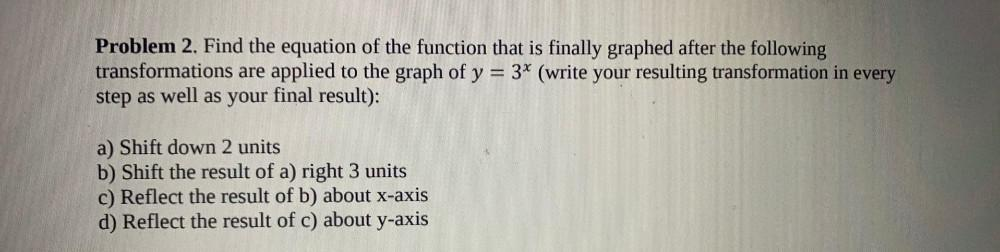Question:

# Find the equation of the function that is finally graphed after the following transformations are applied to the graph of y = 3ˣ (write your resulting transformation in every step as well as your finaFind the equation of the function that is finally graphed after the following transformations are applied to the graph of y = 3ˣ (write your resulting transformation in every step as well as your final result): a) Shift down 2 units b) Shift the result of a) right 3 units c) Reflect the result of b) about x-axis d) Reflect the result of c) about y-axis